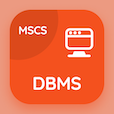Online Engineering Courses

Engineering Mathematics Practice Tests

Learn Online Engineering Mathematics Frequently Asked Questions

# Study guide on First Order Differential Equations PDF

The e-Book Study guide on First Order Differential Equations, Engineering Mathematics Multiple Choice Questions (MCQ Quiz) PDF, download to learn online engineering mathematics courses. Practice Systems Of ODEs Multiple Choice Questions and Answers (MCQs), Engineering Mathematics quiz answers PDF for graduate school interview questions. The eBook Study guide on First Order Differential Equations App Download: differential equations final exam questions with solutions, advanced maths quiz questions with answers, differential equations questions with solutions, differential equations final exam with solutions test prep for online engineering colleges.

The MCQ: Trajectories has critical point which are PDF, "Study guide on First Order Differential Equations" App Download (Free) with 4, 5, 6, and 7 choices for graduate school interview questions. Study study guide on first order differential equations quiz questions, download Amazon eBook (Free Sample) for online engineering programs.

## MCQs: Study guide on First Order Differential Equations

MCQ: Trajectories has critical point which are

A) 4
B) 5
C) 6
D) 7

MCQ: Matrix multiplication is

A) commutative
B) scalar multiplication
C) not commutative
D) logical multiplication

MCQ: A combination of D, multiplication operators and familiar operations such as addition and multiplication is called

A) integral operator
B) difference operator
C) Laplace operator
D) differential operator

MCQ: Operation of writing column as rows and rows as column is termed as

A) derivation
B) integration
C) transportation
D) inversion

MCQ: Derivative of variable entries can be obtained by differentiating

A) whole matrix
B) column
C) row
D) each entry

### Practice Tests: Engineering Mathematics Exam Prep

Download Engineering Math Quiz App, DataBase Management System (MCS) MCQ App, and C++ MCQs App to install for Android & iOS devices. These Apps include complete analytics of real time attempts with interactive assessments. Download Play Store & App Store Apps & Enjoy 100% functionality with subscriptions!